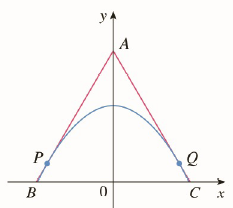Chapter 2.P, Problem 1P

Chapter
Section
Textbook Problem

# Find points P and Q on the parabola y = 1 − x 2 so that the triangle ABC formed by the x-axis and the tangent lines at P and Q is an equilateral triangle. (See the figure.)To determine

To find: points P and Q on the given parabola where tangents form equilateral triangle with x- axis.

Solutions:

The points are:

P-32,14 and Q32,14.

Explanation

1) Concept:

To find points P and Q we have to use concept of equilateral triangle.

Sides AB and AC make equilateral triangle with x axis, they make an angle of 600. This is used for finding slopes of given lines.

Also find slope of the tangent to given curve by using differentiation and solve for x.

We will get one point from these operations.

For second point use symmetry of graph about y – axis.

2) Formula:

i. Slope of tangent =

mtan=tanθ where θ is the angel it makes with positive x-axis.

ii. Slope of tangent to the curve =

dydx.

3) Given:

Tangents through points P and Q to the given curve y=1-x2 forms equilateral triangle ABC with x– axis.

4) Calculations:

As line AB and AC makes angle 60° with x– axis,

Slope of tangent =mtan=tanθ

Slope of line AC is -3, while that of AB is 3 .

By using definition, Slope of tangent to the curve

y= dydx

Therefore dydx= -2x

Hence, for line AC, let x be the x-coordinate of Q then

-2x=-3x=32

Use this in given equation to find value of Y co-ordinate.

y=1-x2=1-322=1-34=14

Therefore, point Q32,14 By using symmetry of the figure about y – axis co-ordinates of point P is -32,14

Conclusion:

The points are:

P-32,14 and Q32,14.

#### The Solution to Your Study Problems

Bartleby provides explanations to thousands of textbook problems written by our experts, many with advanced degrees!

Get Started

#### Differentiate. G(x)=x222x+1

Single Variable Calculus: Early Transcendentals, Volume I

#### In Exercises 6372, evaluate the expression. 71. 21+32

Applied Calculus for the Managerial, Life, and Social Sciences: A Brief Approach

#### In problems 23-58, perform the indicated operations and simplify. 35.

Mathematical Applications for the Management, Life, and Social Sciences

#### Solve the equation for x. 43. |2x| = 3

Single Variable Calculus: Early Transcendentals